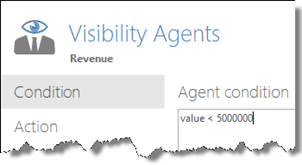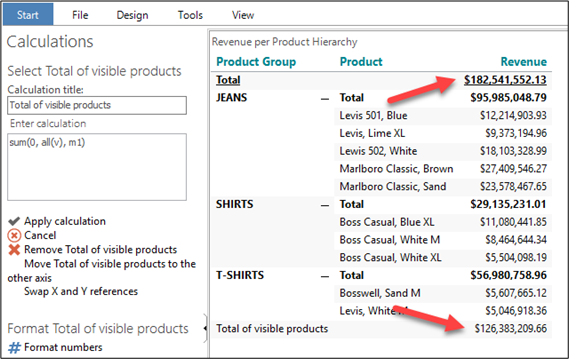# Visibility Modifiers

• Create a new Analysis with a Cross table showing Revenue per Product Hierarchy(Product).
• Use a Visibility Agent to hide products with a Revenue < 5000000.• Add a calculation: Total of visible products.Note: The standard Grand total at the top of the table is the sum of visible and hidden rows, while the calculated total at the bottom of the table is the sum of the visible rows only.

0 out of 0 found this helpful

• Hi!

Is it also possible to use the condition

-x < value < x

To hide all rows with a value between -x and x? I tried but it didn't seem to work.

Jora

• Hi Jora,

You can use boolean AND and OR in your condition. E.g. you can use a condition like this:

value > -x and value < x

BR / Ole

• That helped!
Another question: Is it possible to use an if statement using another field (currency in this case)?

If Currency = A
hide when value > -x and value < x

If Curency = B
value > -y and value < y

• If statements (and calculations in general) can evaluate a condition of a measure value, e.g. value >= x. It cannot evaluate a dimension member string. And I am afraid, in your case, Currency = A or Currency = B will be a reference to a dimension member string.

Of course, if possible, in your data warehouse you could create a measure along with the Currency dimension, e.g. Currency A = 1, Currency B = 2 etc.

Then you would have a measure that you could use in your if statement. The new currency measure has to be part of the object you are working on, but it can be hidden.